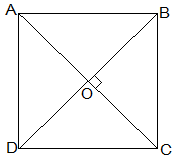Guru

# Show that if the diagonals of a quadrilateral are equal and bisect each other at right angles, then it is a square. Q.5

• 0

Hello sir i want to know the best solution of the question from exercise 8.1of math of Quadrilaterals chapter of class 9th give me the best and easy for solving this question how i solve it of question no. 5 Show that if the diagonals of a quadrilateral are equal and bisect each other at right angles, then it is a square.

Share

1.Given that,

Let ABCD be a quadrilateral and its diagonals AC and BD bisect each other at right angle at O.

To prove that,

The Quadrilateral ABCD is a square.

Proof,

In ΔAOB and ΔCOD,

AO = CO (Diagonals bisect each other)

∠AOB = ∠COD (Vertically opposite)

OB = OD (Diagonals bisect each other)

, ΔAOB ≅ ΔCOD [SAS congruency]

Thus,

AB = CD [CPCT] — (i)

also,

∠OAB = ∠OCD (Alternate interior angles)

⇒ AB || CD

Now,

In ΔAOD and ΔCOD,

AO = CO (Diagonals bisect each other)

∠AOD = ∠COD (Vertically opposite)

OD = OD (Common)

, ΔAOD ≅ ΔCOD [SAS congruency]

Thus,

AD = CD [CPCT] — (ii)

also,

⇒ AD = BC = CD = AB — (ii)

and ∠ADC+∠BCD = 180° (co-interior angles)